python数据分析与可视化——利用Seaborn进行绘图黎明之道1301 0 0

## 利用Seaborn进行绘图

Matplotlib绘图基本模仿MATLAB绘图库，其绘图风格和MATLAB类似。由于MATLAB绘图风格偏古典，因此，Python开源社区开发了Seaborn绘图模块，对Matplotlib进行封装，绘图效果更符合现代人的审美。
Seaborn属于Matplotlib的一个高级接口，使得作图更加容易。在多数情况下使用Seaborn能做出很具吸引力的图，而使用Matplotlib可以制作具有更多特色的图。应该把Seaborn视为iris.csvMatplotlib的补充，而不是替代物。

`import seaborn as sns`

Seaborn通过set函数实现风格设置。
seaborn.set(context=‘notebook’, style=‘darkgrid’, palette=‘deep’, font=‘sans-serif’, font_scale=1, color_codes=True, rc=None)

### 利用seaborn.set进行风格设置

``````import seaborn as sns
import numpy as np
import matplotlib as mpl
import matplotlib.pyplot as plt
def sinplot(flip=2):
x = np.linspace(0,20, 50)
for i in range(1,5):
plt.plot(x, np.cos(x + i * 0.8) * (9 - 2*i) * flip)
sinplot() ``````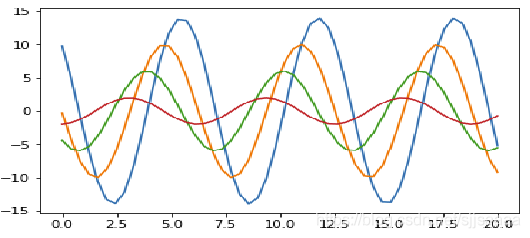``````sns.set(style='darkgrid',font_scale=1.5)
sinplot() ``````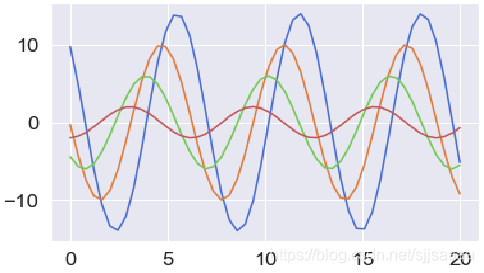``````sns.set()
sinplot() ``````

Seaborn有五个预设好的主题： darkgrid， whitegrid，dark，white，和 ticks，默认为darkgrid。

``````sns.set_style("whitegrid")
sinplot() ``````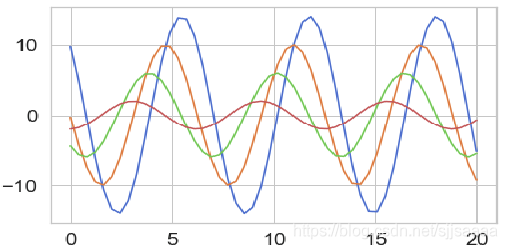Seaborn将matplotlib的参数划分为两个独立的组合。第一组是设置绘图的外观风格的，第二组主要将绘图的各种元素按比例缩放的，以至可以嵌入到不同的背景环境中。控制这些参数的接口主要有两对方法：

``````sns.set_style("whitegrid")
sinplot() ``````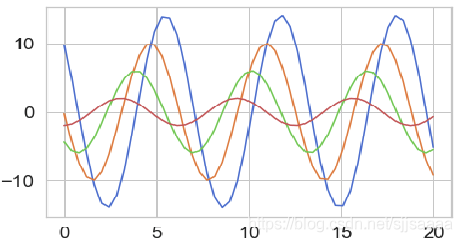``````sinplot()
sns.despine() ``````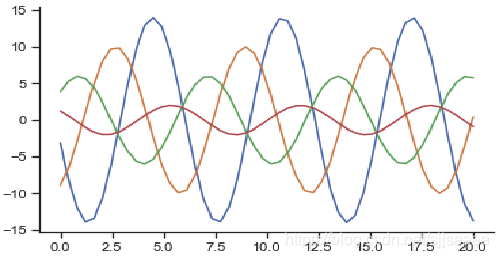despine（）方法中可以利用offset参数讲轴线进行偏置，另外，当刻度没有完全覆盖整个坐标轴的的范围时，利用trim参数限制已有坐标轴的范围。

``````sinplot()
sns.despine(offset=20, trim=True) ``````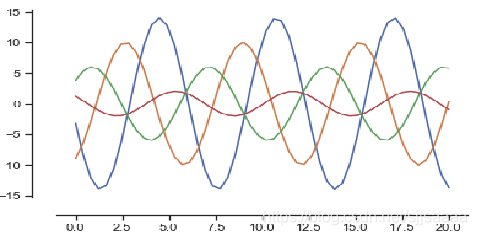``````sinplot()
sns.despine(left=True) ``````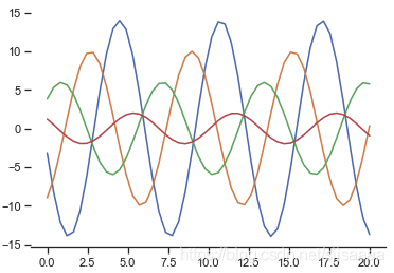``````sinplot()
sns.set(style='whitegrid',palette='muted',color_codes=True)
sns.despine(left=True,bottom=True) ``````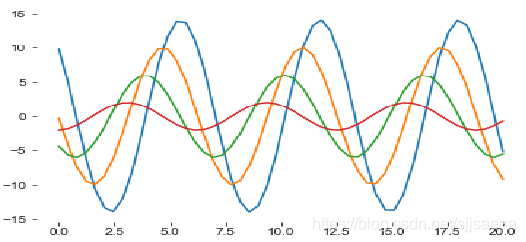``````with sns.axes_style("darkgrid"):
plt.subplot(2,1,1)
sinplot( )
plt.subplot(2,1, 2)
sinplot(-1) ``````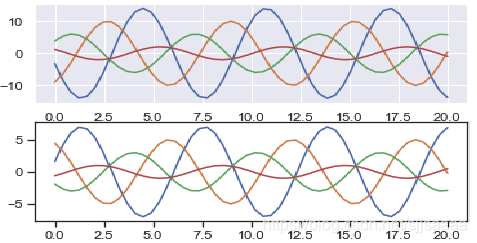seaborn中通过set_context()设置缩放参数，预设的参数有paper, notebook, talk, poster。默认为notebook。

``````sns.set_style("darkgrid", {"axes.facecolor": ".9"})
sinplot() ``````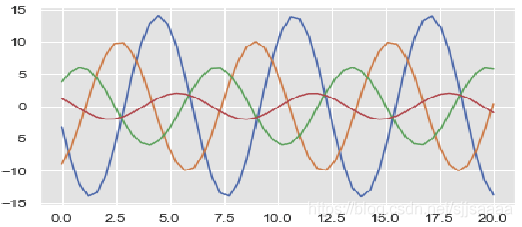``````sns.set_context("paper")
sinplot() ``````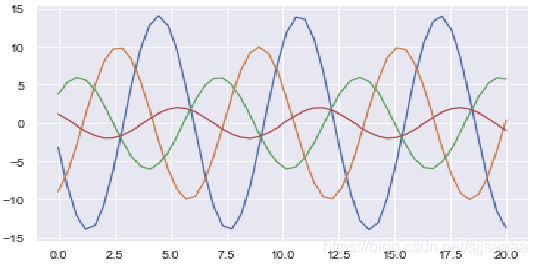``````sns.set_context("notebook", font_scale=1.8, rc={"lines.linewidth": 1.5})
sinplot() ``````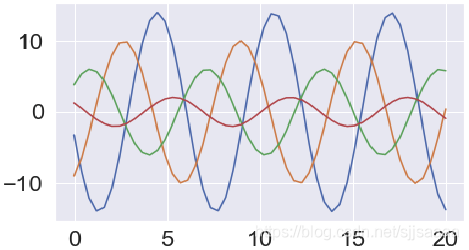### 常用的绘图

#### 直方图和密度曲线图

Seaborn中利用distplot( )和 kdeplot( )绘制直方图和密度曲线图，distplot( )为hist加强版，默认情况下绘制一个直方图，并嵌套一个对应的密度图。

``````import matplotlib.pyplot as plt
import seaborn as sns
import numpy as np
sns.set(palette="muted",color_codes=True)
rs=np.random.RandomState(10)
d=rs.normal(size=100)
f,axes=plt.subplots(2, 2, figsize=(7, 7), sharex=True)
sns.distplot(d, kde=False, color="b", ax=axes[0,0])
sns.distplot(d, hist=False, rug=True, color="r", ax=axes[0,1])
sns.distplot(d, color="m", ax=axes[1,1])
plt.show() ``````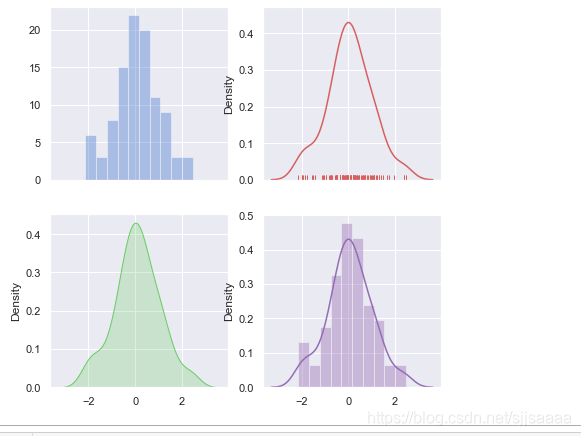#### 散点图

``````sns.set(style='white',color_codes=True) #设置样式
sns.stripplot(x=df_iris['Species'],y= df_iris['Petal.Width'],data=df_iris)
sns.despine()   #去坐标轴 ``````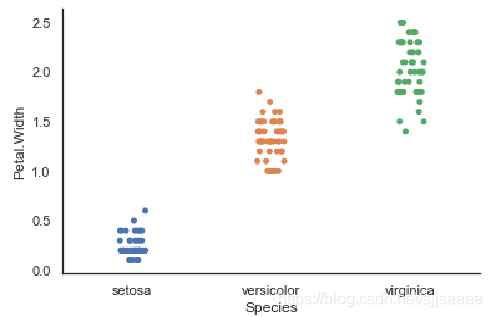``````sns.swarmplot(x=df_iris['Species'],y= df_iris['Petal.Width'],data=df_iris)
sns.despine()   #去坐标轴 ``````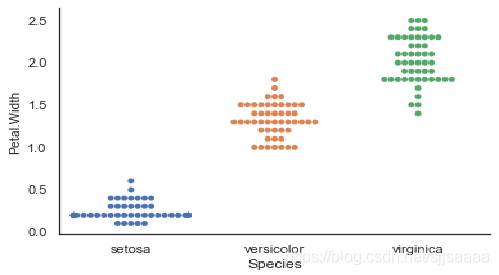#### 箱型图

``````df_iris=pd.read_csv('D:\dataset\iris.csv')
sns.boxplot(x=df_iris['Species'],y = df_iris['Petal.Width'])
plt.show() ``````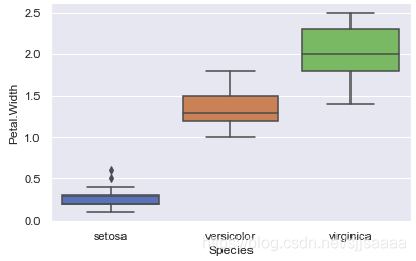``````df_iris=pd.read_csv('D:\dataset\iris.csv')
sns.set(style="ticks")
sns.boxplot(x=df_iris['Species'],y = df_iris['Petal.Width'])
plt.show() ``````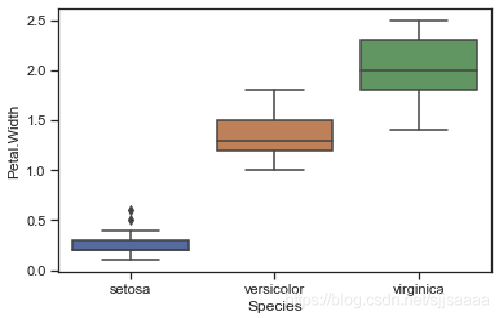#### pairplot

``seaborn.pairplot(data, hue=None, hue_order=None, palette=None, vars=None, x_vars=None, y_vars=None, kind='scatter', diag_kind='auto', markers=None, height=2.5, aspect=1, dropna=True, plot_kws=None, diag_kws=None, grid_kws=None, size=None) ``

pairplot主要展现的是变量两两之间的关系（线性或非线性，有无较为明显的相关关系）

``````df_iris=pd.read_csv('D:\dataset\iris.csv')
sns.set(style="ticks")
g = sns.pairplot(df_iris,vars=['Sepal.Length', 'Petal.Length']) ``````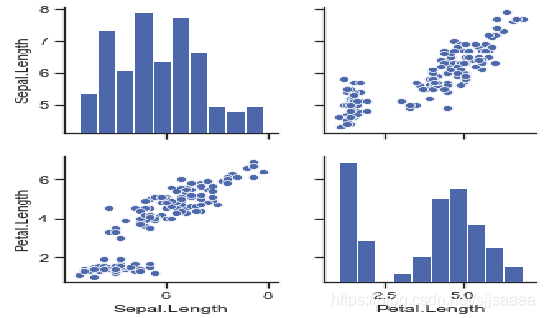#### 小提琴图

``````sns.set_style("whitegrid")
ax = sns.violinplot(x=df_iris['Petal.Length']) ``````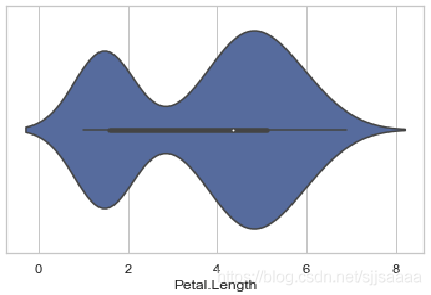#### 柱状图

``````df_iris=pd.read_csv('D:\dataset\iris.csv')
sns.barplot(x=df_iris['Species'],y=df_iris['Petal.Length'],data=df_iris) ``````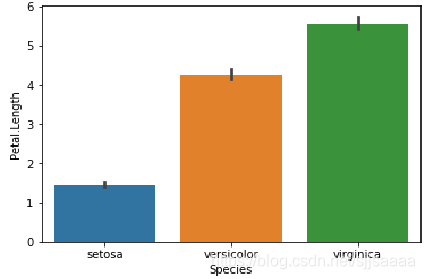``sns.countplot(x='Species',data=df_iris) ``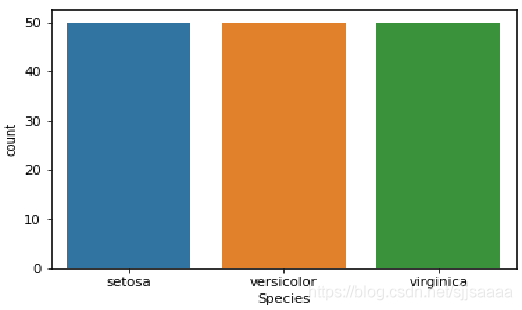#### 多变量图

``sns.jointplot(x='Petal.Length',y='Petal.Width' ,data=df_iris) ``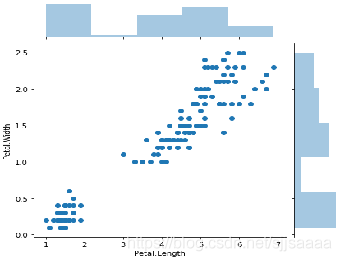``ointplot(x='Petal.Length',y='Petal.Width' ,data=df_iris,kind='kde') ``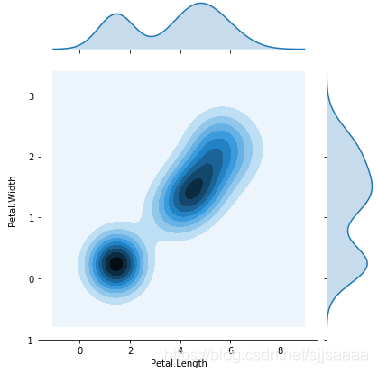#### 回归图

``sns.regplot(x='Petal.Length',y='Petal.Width' ,data=df_iris) ``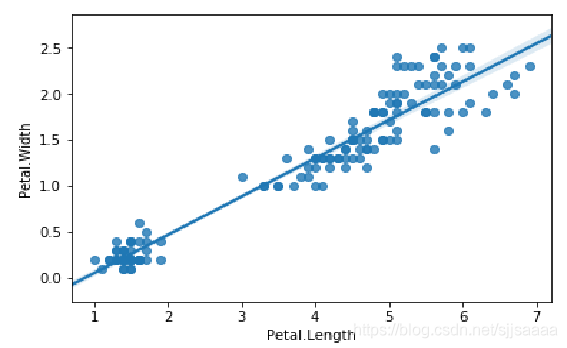### 相关推荐

15. Python 程序运行速度如何提高十倍？第一遍滚雪球学 Python 收工

python数据分析与可视化——利用Seaborn进行绘图

&ensp;&ensp;&ensp;  近几年来，python的热度一直特别火！大学期间，也进行了一番深入学习，毕业后也曾试图把python作为自己的职业方向，虽然没有如愿成为一名python工程师，但掌握了python，也让我现如今的工作开展和职业发展更加得心应手。这篇文章主要与大家分享一下自己在python爬虫方面的收获与见解。 &ensp;&ensp;
plotnine: Python版的ggplot2作图库
R语言的ggplot2绘图能力超强，python虽有matplotlib，但是语法臃肿，使用复杂，入门极难，seaborn的出现稍微改善了matplotlib代码量问题，但是定制化程度依然需要借助matplotlib，使用难度依然很大。 而且咱们经管专业学编程语言，一直有一个经久不衰的问题\“学数据分析，到底选择R还是Python”。通过plotnine这
5 行 Python 代码调用电脑摄像头
**前提：** 确保 python 中安装了 opencv-python 模块。如果没有安装，可以参考：[https://pypi.org/project/opencv-python/](https://www.oschina.net/action/GoToLink?url=https%3A%2F%2Fpypi.org%2Fproject%2Fopencv
FreeBSD python安装MySQL
fetch https://pypi.python.org/packages/source/M/MySQL-python/MySQL-python-1.2.4.zip unzip MySQL-python-1.2.4.zip cd MySQL-python-1.2.4 python setup.py install ln -s /usr/loca
Jenkins Python Plugin获取环境变量
Linux 下的 Python 多版本管理（pyenv）
#简介 提到 Python 环境管理，更多人可能会首先想到 virtualenv。但与用于创建独立包环境的 virtualenv 不同，pyenv 的作用仅限于维护不同版本的 Python。它的使用不依赖于 Python，是一个简单、独立的纯 shell 脚本工具。pyenv 也以 [pyenv-virtualenv](https://www.oschin
Python 3 教程
Python 3 教程 =========== ![python3](https://www.runoob.com/wp-content/uploads/2014/05/python3.png) Python 的 3.0 版本，常被称为 Python 3000，或简称 Py3k。相对于 Python 的早期版本，这是一个较大的升级。为了不带入过多的累赘，
Python 3.8 官网文档（中文版附下载）
![](https://oscimg.oschina.net/oscnet/b5c848a0d97764f322d36cce2d2a8d80f4b.jpg) 文档解释了 Python 3.8 相比 3.7 的新增特性。本参考手册描述了 Python 的语法和“核心语义”。本参考是简洁的，但试图做到准确和完整。 这份库参考
Python MySQLdb 循环插入execute与批量插入executemany性能分析
Python MySQLdb 循环插入execute与批量插入executemany性能分析 ============================================== 用Python连接MySQL数据库时，会用到MySQLdb库，这里下载↓↓↓ https://pypi.python.org/pypi/MySQL-python/ 这
Python中的参数传递与解析
Python传递命令行参数 ============= Python的命令行参数传递和C语言类似，都会把命令行参数保存到argv的变量中。对于python而言，argv是sys模块中定义的一个list。与C语言不同的是，python中并没有定义argc，要获得参数的个数，需要使用len(sys.argv) 当用户使用'python -c "command
Python图表数据可视化Seaborn：2. 分类数据可视化
1\. 分类数据可视化 - 分类散点图 =================== **stripplot( ) / swarmplot( )** sns.stripplot(x="day",y="total_bill",data=tips,jitter = True, size = 5, edgecolor = 'w',linewidth=1,ma
Seaborn学习02：折线图（多坐标）

【Python学习教程】常用的8个Python数据可视化库！
Python是一门非常优秀的编程语言，被广泛的应用于各个领域，它不仅简单、易懂、语法清晰，还拥有各种各样的库，让我们的工作更高效、更便捷。今天为大家分享8个Python库，有了它们之后，数据可视化超轻松，快来学习一下吧。　　数据可视化是展示数据、理解数据的有效手段，常用的Python数据可视化库如下：　　1.Matplotlib 第一个Python可视化库
HelloWorld开发者社区 - 开发者专属的技术社区